# Texas Go Math Grade 5 Unit 3 Answer Key Algebraic Reasoning

Refer to our Texas Go Math Grade 5 Answer Key Pdf to score good marks in the exams. Test yourself by practicing the problems from Texas Go Math Grade 5 Unit 3 Answer Key Algebraic Reasoning.

## Texas Go Math Grade 5 Unit 3 Answer Key Algebraic Reasoning

Show What You Know

Check your understanding of important skills.

Question 1.
Since 3 × _______ = 12
then 12 ÷ 3 = _________.
Since 3 × 4 = 12
then 12 ÷ 3 = 4.
Explanation:
Multiplication is also known as the inverse (or “opposite”) of Division. For example, since , then 12 ÷ 3 = 4.  As such, knowing the multiplication tables can be helpful with division.

Question 2.
Since 48 ÷ 6 = _______.
then _______ × 6 = 48.
Since 48 ÷ 6 = 8.
then 8 × 6 = 48.
Explanation:
Division is a basic algebraic operation where we split a number into equal parts of groups. This is expressed as    48 ÷ 6 = 8. Division is also known as the inverse (or “opposite”) of Multiplication. For example, since 48 ÷ 6 = 8 , then 8 x 6 = 48.

Question 3.
Since 7 × _______ = 70
then 70 ÷ 7 = _________.
Since 7 × 10 = 70
then 70 ÷ 7 = 10.
Explanation:
Multiplication is also known as the inverse (or “opposite”) of Division. For example, since , then
70 ÷ 7 = 10.  As such, knowing the multiplication tables can be helpful with division.

Question 4.
Since 54 ÷ 9 = _______.
then _______ × 9 = 54.
Since 54 ÷ 9 = 6.
then 6 × 9 = 54.
Explanation:
Division is a basic algebraic operation where we split a number into equal parts of groups. This is expressed as    54 ÷ 9 = 6. Division is also known as the inverse (or “opposite”) of Multiplication. For example, since 54 ÷ 9 = 6 , then 6 x 9 = 54.

Missing Factors Find the missing factor.

Question 5.
4 × ______ = 24
4 x 6 = 24
Explanation:
The missing factor in the above multiplication operation is 6.

Question 6.
6 × _______ = 36
6 x 6 = 36
Explanation:
The missing factor in the above multiplication operation is 6.

Question 7.
________ × 9 = 63
7 x 9 = 63
Explanation:
The missing factor in the above multiplication operation is 7.

Question 8.
_________ × 5 = 40
8 x 5 = 40
Explanation:
The missing factor in the above multiplication operation is 8.

Question 9.
_________ × 8 = 16
2 x 8 = 16
Explanation:
The missing factor in the above multiplication operation is 2.

Question 10.
11 × ________ = 88
11 x 8 = 88
Explanation:
The missing factor in the above multiplication operation is 8.

Area Write the area of each figure.

Question 11.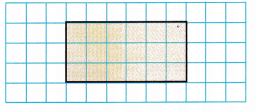____________ square unitsArea of the rectangle = length x breadth
Area of the rectangle = 3 x 6 = 18 square units
The area is 18 square units.
Explanation:
In the above figure we can observe that some area is covered. The covered are is in the shape of rectangle. We have to calculate the area of the rectangle for the above figure. The covered area is 18 square units.

Question 12.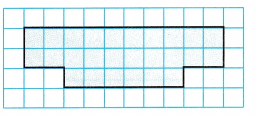____________ square unitsArea is 26 square units.
Explanation:
In the above figure we can observe that some area is covered. We have to calculate the area for the above figure. The covered area is 26 square units.

Question 13.____________ square unitsArea of the rectangle = length x breadth
Area of the rectangle = 1 x 7 = 7 square units
Area is 7 square units.
Explanation:
In the above figure we can observe that some area is covered. The covered area is in the shape of rectangle. We have to calculate the area of the rectangle for the above figure. The covered area is 7 square units.

Question 14.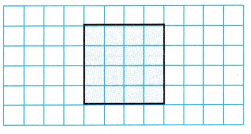____________ square unitsArea of square = side x side
Area of square = 4 x 4 = 16 square units
Area is 16 square units.
Explanation:
In the above figure we can observe that some area is covered. The covered area is in the shape of square. We have to calculate the area of the square for the above figure. The covered area is 16 square units.

Vocabulary Builder

Visualize It

Match the ✓ words with their examples.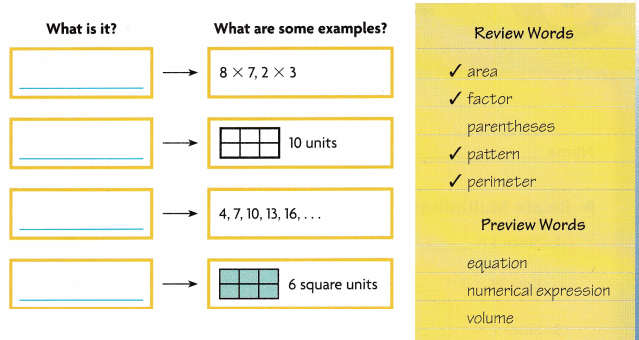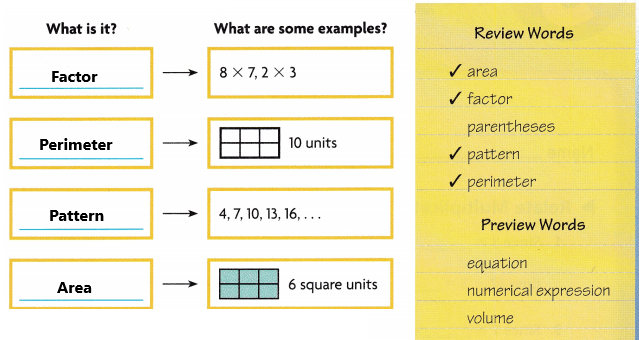Understand Vocabulary

Complete the sentences with the words.

Question 1.
A ___________ is an ordered set of numbers or objects.
A pattern is an ordered set of numbers or objects.

Question 2.
___________ is the distance around a closed plane figure.
Perimeter is the distance around a closed plane figure.

Question 3.
A ___________ is a mathematical phrase that has numbers and operation signs but does not have an equal sign.
A numerical expression is a mathematical phrase that has numbers and operation signs but does not have an equal sign.

Question 4.
___________ is the measure of the number of unit squares.
Area is the measure of the number of units squares.

Question 5.
An ___________ is an algebraic or numerical sentence that shows that two quantities are equal.
An equation is an algebraic or numerical sentence that shows that two quantities are equal.

Question 6.
___________ are the symbols used to show which operation or operations in an expression should be done first.
Parentheses are the symbols used to show which operation or operations in an expression should be done first.

Question 7.
___________ is the measure of the space a solid figure occupies.
Volume is the measure of the space a solid figure occupies.

Question 8.
A ___________ is a number multiplied by another number to find a product.
A factor is a number multiplied by another number to find a product.

Reading When you read a story, you interpret the words, sentences, and paragraphs. When you read math, you have to go beyond words. Often you must analyze graphs and tables to get the information you need.

Read the problem. Study the input/output table.

Vann works part-time for the Transit Authority. He earns \$12 an hour. The input column of the input/output table shows the number of hours, x, that he works. The output column shows how much he earns, y. What is the rule for the table?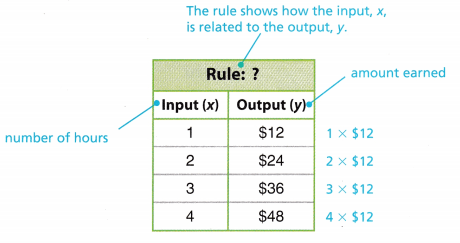Writing Keith drives a limo to the airport. He can take 4 passengers at a time. Write a problem that can be answered by using this information and an input/output table. Ask a classmate to solve the problem.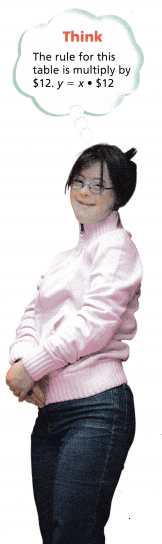Make It Even
Object of the Game Make an expression equal to an even number.

Materials
Number/Symbol Cards: 3 sets labeled 0—9, 2 sets labeled +, —
Number of Players 2

Set Up
Give each player a set of symbol cards. Shuffle the number cards and place them face down in a stack.

How to Play

(1) Each player draws 3 number cards from the stack. Players use the number cards and one or two of his or her symbol cards to make an expression.
(2) A player as score is the value of the expression. If the value is an even number, the score is doubled.
(3) Return all the cards to the deck and shuffle. Repeat steps 1-2.
(4) The player with the highest score after 4 rounds wins.